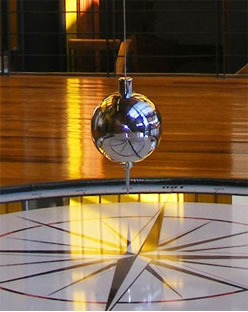The Foucault pendulum and demonstration of the Earth´s rotationIf one looks at a pendulum, standing up and fixed to the Earth, a slight displacement of the point where the pendulum reaches its maximum aperture will be observed after several oscillations.If our planet was stationary in space, we would observe that the pendulum’s oscillation plane does not change. This is the argument used by Foucault to argue that his experiment finally demonstrated the Earth’s rotation.Let us first consider a pendulum oscillating exactly in the North Pole. Given that the Earth’s rotation axis goes through both Poles, the ground rotates in a counter clockwise direction with respect to distant stars. Since the pendulum’s oscillation plane does not change with respect to these stars, an observer on the ground will see how the pendulum’s oscillation plane makes a complete turn in a clockwise direction in that same time. On the other hand, an observer on Earth, in the South Pole, will observe that the pendulum’s oscillation plane will make a complete turn in the same time, but in an anti-clockwise direction. What happens if we run the experiment exactly in the Equator?Unlike what happens in the Poles, the Earth’s rotation axis in the Equator is parallel to the ground; consequently, the ground does not turn with respect to the axis. Thus, the observer standing still on the ground,always sees the pendulum oscillating on the same plane. The Earth’s rotation cannot be detected using a pendulum in the Equator.Now let us consider a place between the South Pole and the Equator; for example, a location in Chile. The base of the pendulum, on the ground, is neither parallel nor perpendicular to the Earth’s rotation axis; in consequence, the ground will rotate, but slower than in the Poles. Thus, the observer fixed on Earth will see that the pendulum’s oscillation plane will turn in an anti-clockwise direction (because he/she is in the Southern Hemisphere), but it will take longer to make a complete turn.The mathematical description of the movement of the pendulum in any latitude is somewhat complex. It establishes the following formula to estimate the time (in hours) required by the pendulum to make a complete turn: T=24/sen(A), where A is the latitude of the pendulum’s location. For the latitude of Valdivia, T is approximately 36 hours. In consequence, the oscillation plane turns around 10 degrees per hour.